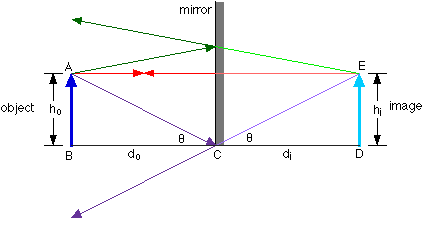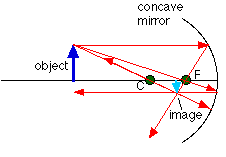The reflection of light

7-25-00

Sections 23.1 - 23.3

Rays and wave fronts

Light is a very complex phenomenon, but in many situations its behavior can be understood with a simple model based on rays and wave fronts. A ray is a thin beam of light that travels in a straight line. A wave front is the line (not necessarily straight) or surface connecting all the light that left a source at the same time. For a source like the Sun, rays radiate out in all directions; the wave fronts are spheres centered on the Sun. If the source is a long way away, the wave fronts can be treated as parallel lines.

Rays and wave fronts can generally be used to represent light when the light is interacting with objects that are much larger than the wavelength of light, which is about 500 nm. In particular, we'll use rays and wave fronts to analyze how light interacts with mirrors and lenses.

The law of reflection

Objects can be seen by the light they emit, or, more often, by the light they reflect. Reflected light obeys the law of reflection, that the angle of reflection equals the angle of incidence.For objects such as mirrors, with surfaces so smooth that any hills or valleys on the surface are smaller than the wavelength of light, the law of reflection applies on a large scale. All the light travelling in one direction and reflecting from the mirror is reflected in one direction; reflection from such objects is known as specular reflection.

Most objects exhibit diffuse reflection, with light being reflected in all directions. All objects obey the law of reflection on a microscopic level, but if the irregularities on the surface of an object are larger than the wavelength of light, which is usually the case, the light reflects off in all directions.

Plane mirrors

A plane mirror is simply a mirror with a flat surface; all of us use plane mirrors every day, so we've got plenty of experience with them. Images produced by plane mirrors have a number of properties, including:

1. the image produced is upright
2. the image is the same size as the object (i.e., the magnification is m = 1)
3. the image is the same distance from the mirror as the object appears to be (i.e., the image distance = the object distance)
4. the image is a virtual image, as opposed to a real image, because the light rays do not actually pass through the image. This also implies that an image could not be focused on a screen placed at the location where the image is.

A little geometry

Dealing with light in terms of rays is known as geometrical optics, for good reason: there is a lot of geometry involved. It's relatively straight-forward geometry, all based on similar triangles, but we should review that for a plane mirror.

Consider an object placed a certain distance in front of a mirror, as shown in the diagram. To figure out where the image of this object is located, a ray diagram can be used. In a ray diagram, rays of light are drawn from the object to the mirror, along with the rays that reflect off the mirror. The image will be found where the reflected rays intersect. Note that the reflected rays obey the law of reflection. What you notice is that the reflected rays diverge from the mirror; they must be extended back to find the place where they intersect, and that's where the image is.Analyzing this a little further, it's easy to see that the height of the image is the same as the height of the object. Using the similar triangles ABC and EDC, it can also be seen that the distance from the object to the mirror is the same as the distance from the image to the mirror.

Spherical mirrors

Light reflecting off a flat mirror is one thing, but what happens when light reflects off a curved surface? We'll take a look at what happens when light reflects from a spherical mirror, because it turns out that, using reasonable approximations, this analysis is fairly straight-forward. The image you see is located either where the reflected light converges, or where the reflected light appears to diverge from.

A spherical mirror is simply a piece cut out of a reflective sphere. It has a center of curvature, C, which corresponds to the center of the sphere it was cut from; a radius of curvature, R, which corresponds to the radius of the sphere; and a focal point (the point where parallel light rays are focused to) which is located half the distance from the mirror to the center of curvature. The focal length, f, is therefore:

focal length of a spherical mirror : f = R / 2

This is actually an approximation. Parabolic mirrors are really the only mirrors that focus parallel rays to a single focal point, but as long as the rays don't get too far from the principal axis then the equation above applies for spherical mirrors. The diagram shows the principal axis, focal point (F), and center of curvature for both a concave and convex spherical mirror.Spherical mirrors are either concave (converging) mirrors or convex (diverging) mirrors, depending on which side of the spherical surface is reflective. If the inside surface is reflective, the mirror is concave; if the outside is reflective, it's a convex mirror. Concave mirrors can form either real or virtual images, depending on where the object is relative to the focal point. A convex mirror can only form virtual images. A real image is an image that the light rays from the object actually pass through; a virtual image is formed because the light rays can be extended back to meet at the image position, but they don't actually go through the image position.

Ray diagrams

To determine where the image is, it is very helpful to draw a ray diagram. The image will be located at the place where the rays intersect. You could just draw random rays from the object to the mirror and follow the reflected rays, but there are three rays in particular that are very easy to draw.

Only two rays are necessary to locate the image on a ray diagram, but it's useful to add the third as a check. The first is the parallel ray; it is drawn from the tip of the object parallel to the principal axis. It then reflects off the mirror and either passes through the focal point, or can be extended back to pass through the focal point.

The second ray is the chief ray. This is drawn from the tip of the object to the mirror through the center of curvature. This ray will hit the mirror at a 90° angle, reflecting back the way it came. The chief and parallel rays meet at the tip of the image.

The third ray, the focal ray, is a mirror image of the parallel ray. The focal ray is drawn from the tip of the object through (or towards) the focal point, reflecting off the mirror parallel to the principal axis. All three rays should meet at the same point.A ray diagram for a concave mirror is shown above. This shows a few different things. For this object, located beyond the center of curvature from the mirror, the image lies between the focal point (F) and the center of curvature. The image is inverted compared to the object, and it is also a real image, because the light rays actually pass through the point where the image is located.

With a concave mirror, any object beyond C will always have an image that is real, inverted compared to the object, and between F and C. You can always trade the object and image places (that just reverses all the arrows on the ray diagram), so any object placed between F and C will have an image that is real, inverted, and beyond C. What happens when the object is between F and the mirror? You should draw the ray diagram to convince yourself that the image will be behind the mirror, making it a virtual image, and it will be upright compared to the object.